# 线性方程组### 例子：你和马赛跑你： d = 0.2t 马： d = 0.5(t−6)## 求解

### 例子：解这两个方程：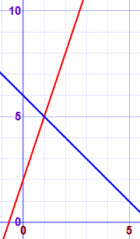• x + y = 6
• −3x + y = 2

x + y − (−3x + y) = 6 − 2

x + y + 3x − y = 6 − 2
4x = 4
x = 1

x=1 是在两条线上。

x + y = 6
1 + y = 6
y = 5

x = 1 和 y = 5

## 线性方程

 线性方程 可以使 2 维的…… （例如 x 和 y）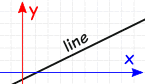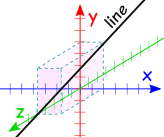……或 3 维 （例如 x、y 和 z）…… ……或 4 维……甚至更高！ （但不能画出来）## 很多变量

### 例子：3 个方程，3 个变量

 2x + y − 2z = 3 x − y − z = 0 x + y + 3z = 12

• 2 个方程，3 个变量，
• 6 个方程，4 个变量，
• 9,000 个方程，567 个变量，
• 等等

## 解

• 无（零）
• 个解
• 无穷多个解## 独立

"独立" 的意思是每个方程都含有新信息。

• x + y = 3
• 2x + 2y = 6

## 方程在哪里为真

### 例子：你与马• 在 t=5 和 d=1，方程是 的 （d = 0.2t 吗？是，1 = 0.2×5 是对的）
• 在 t=5 和 d=3，方程是 不对 的 （d = 0.2t 吗？不，3 = 0.2×5 是不对的

。。。。。线性方程组的另一个名字是 "联立线性方程"

## 用代数来解

### 例子：你与马

• d = 0.2t
• d = 0.5(t-6)

d = 0.2t = 0.5(t-6)

 展开 0.5(t-6)： 0.2t = 0.5t - 3 每边减 0.5t： -0.3t = -3 每边除以 -0.3： t = -3/-0.3 = 10分钟 知道你在什么时候被马赶上了！ 知道 t，我们便可以求 d： d = 0.2t = 0.2×10 = 2 km

t = 10分钟 d = 2 km

• 代入法
• 消元法

## 代入法

• 把一个方程写成 "变量 = ……" 的形式
• 在其他的方程中替代（代入）那个变量。
• 其他的方程
• （如有需要，重复以上步骤）

2 个方程，2 个变量 的例子：

### 例子：

• 3x + 2y = 19
• x + y = 8

• 3x + 2y = 19
• y = 8 - x

• 3x + 2(8 - x) = 19
• y = 8 - x

• 3x + 16 - 2x = 19
• y = 8 - x

3x-2x = x

• x + 16 = 19
• y = 8 - x

• x = 3
• y = 8 - x

• x = 3
• y = 8 - 3 = 5

x = 3y = 5

## 代入法：3 个方程，3 个变量

### 例子：

• x + z = 6
• z - 3y = 7
• 2x + y + 3z = 15

 x + z = 6 - 3y + z = 7 2x + y + 3z = 15

 x = 6 - z - 3y + z = 7 2x + y + 3z = 15

（只有第三个方程有变量 x ）

 x = 6 - z - 3y + z = 7 2(6-z) + y + 3z = 15

2(6-z) + y + 3z = 15 简化成 y + z = 3

 x = 6 - z - 3y + z = 7 y + z = 3

 x = 6 - z - 3y + z = 7 z = 3 - y

 x = 6 - z - 3y + 3 - y = 7 z = 3 - y

-3y + (3-y) = 7 简化成 -4y = 4，就是 y = -1

 x = 6 - z y = -1 z = 3 - y

 x = 6 - z y = -1 z = 4

 x = 2 y = -1 z = 4

x = 2y = -1z = 4

## 消元法

"消" 的意思是移除：消元法就是把变量逐个移除，直至只剩下一个。

• 把方程乘以常数（除了零以外），
• 把两个方程相加（或相减）### 为什么可以把方程相加？

x − 5 = 3
5 = 5

x − 5 + 5 = 3 + 5
x = 8

### 例子：

• 3x + 2y = 19
• x + y = 8

 3x + 2y = 19 x + y = 8

 3x + 2y = 19 2x + 2y = 16

 x = 3 2x + 2y = 16

 x = 3 x + y = 8

 x = 3 y = 5

x = 3y = 5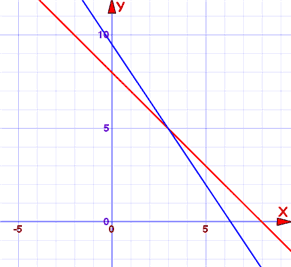### 例子：

• 2x - y = 4
• 6x - 3y = 3

 2x - y = 4 6x - 3y = 3

 6x - 3y = 12 6x - 3y = 3

 0 - 0 = 9 6x - 3y = 3

0 - 0 = 9 ？？？

 两个方程的图是平行线：### 例子：

• 2x - y = 4
• 6x - 3y = 12

 2x - y = 4 6x - 3y = 12

 6x - 3y = 12 6x - 3y = 12

 0 - 0 = 0 6x - 3y = 3

0 - 0 = 0

……这是因为它们其实是同一个方程…….

……所以有无穷多的解

 是同一条线：• 无（零）
• 个解
• 无穷多个解

## 消元法：3 个方程，3 个变量

• 先消除 x（从第二个和第三个方程，顺序）
• 然后从第三个方程中消除 y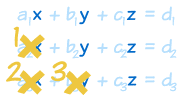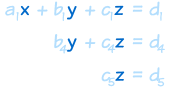9代入 z 来求 y，然后代入 zy 来求 x）：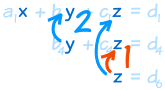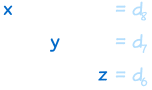### 例子：

• x + y + z = 6
• 2y + 5z = -4
• 2x + 5y - z = 27

 x + y + z = 6 2y + 5z = -4 2x + 5y - z = 27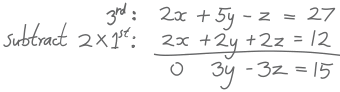x + y + z = 6 2y + 5z = -4 3y - 3z = 15

。。。。但我们可以不用分数，如果我们：

• 把第三个方程乘以 2
• 把第二个方程乘以 3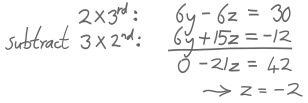x + y + z = 6 2y + 5z = -4 z = -2

 x + y + z = 6 y = 3 z = -2

x+y+z=6 变成 x+3-2=6，所以 x=6-3+2=5

 x = 5 y = 3 z = -2

x = 5y = 3z = -2

## 忠告

• 若只有几个方程，代入法往往会比较容易（例如，2 个方程，有时 3 个方程）
• 若有很多方程，消元法就比较容易